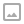## 「CF875E」Delivery Club-二分+贪心

·  ✏️ About  681 words  ·  ☕ 2 mins read · 👀... views

Codeforces

## 代码

  1 2 3 4 5 6 7 8 9 10 11 12 13 14 15 16 17 18 19 20 21 22 23 24 25 26  #include using namespace std; const int MAXN = 110000; int n,s1,s2,x[MAXN]; bool check(int M){ int L = x[n]-M,R = x[n]+M; for(int i = n-1;i>=1;--i){ if(L <= x[i] && x[i] <= R) L = x[i] - M,R = x[i] + M; else L = max(L,x[i]-M),R = min(R,x[i]+M); } return (L <= s1 && s1 <= R) || (L <= s2 && s2 <= R); } int main(){ scanf("%d %d %d",&n,&s1,&s2); for(int i = 1;i<=n;i++) scanf("%d",&x[i]); int L = abs(s1-s2),R = 1e9; while(L!=R){int mid = (L+R)/2; if(check(mid)) R = mid; else L = mid+1; } printf("%d\n",L); }WRITTEN BY
cqqqwq
A student in Computer Science.